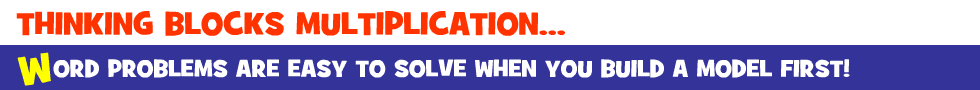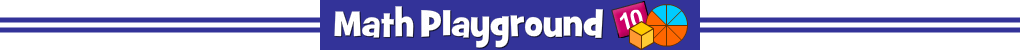Thinking Blocks Multiplication - Learning Connections
Essential Skills
Problem Solving - model and solve word problems

Common Core Connection for Grades 3 and 4
Solve word problems involving equal groups, arrays and measurement quantities.
Use equations with a symbol for the unknown number to represent the problem.
Solve two-step word problems using the four operations.
Represent problems using equations with a letter standing for the unknown quantity.

More Math Games to Play